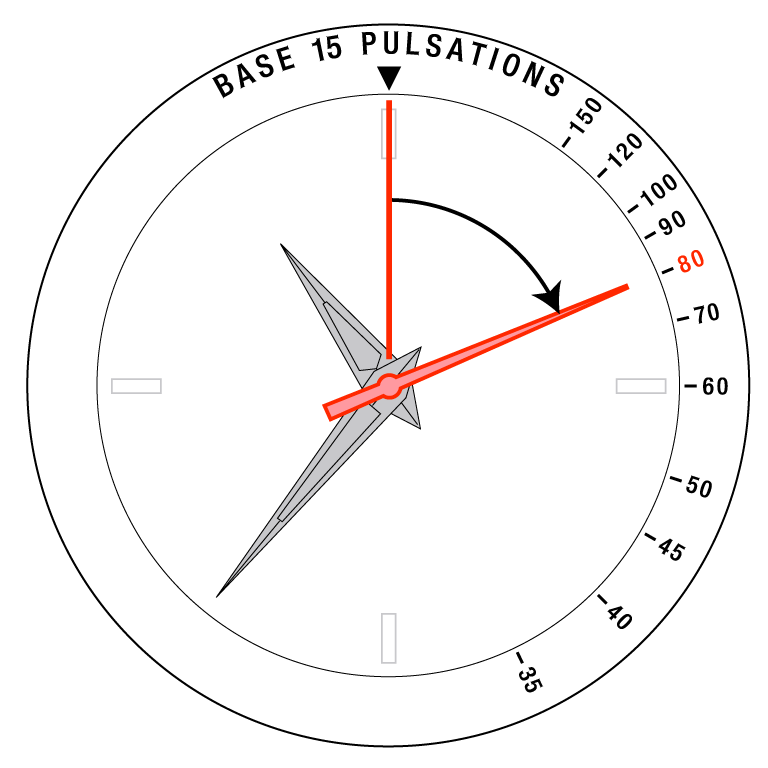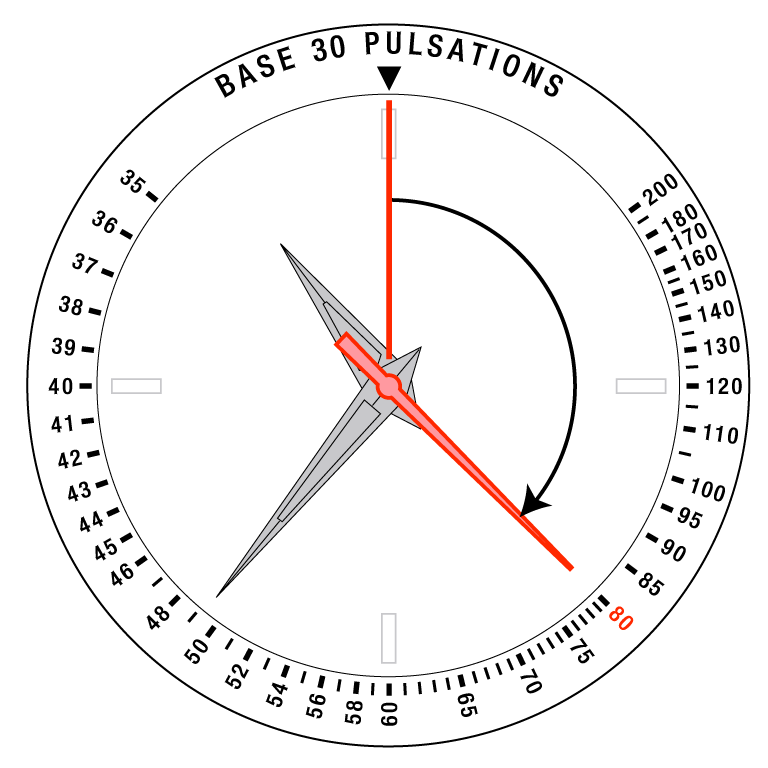## How to use the pulse meter

You can use the pulse meter to measure an approximate pulse rate (number of pulses per minute) in shorter time.

<When measuring with the chronograph>

1) Start counting pulses as you start the chronograph.

2) Stop the chronograph as you counted a specified number of pulses.

•You can find the number of pulses to count on the bezel or the dial.

3) The value which the chronograph hand is pointing is the approximate pulse rate per minute.

•On the examples, the pulse rate per minute is about 80.

<When measuring with the second hand>

1) Start counting pulses as the second hand points 12 o’clock.

2) The value which the second hand pointed as you counted a specified number of pulses is the approximate pulse rate per minute.

•You can find the number of pulses to count on the bezel or the dial.

•On the examples, the pulse rate per minute is about 80.

Example of the 15-pulse counting typeExample of the 30-pulse counting type